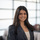# Window Functions in MySQL (with Python!)

I've come across a few use cases where customers would like to apply a SQL window function, but are limited to using a version of MySQL that does not support window functions. Traditionally our recommendations would have been one of the following:

1. Cache the underlying dataset so we could leverage Redshift syntax to write the query (and therefore, take advantage of window functions)
2. Use a different approach for the query that avoids window functions altogether

Thanks to Periscope's built-in Python Integration, a third option now exists - use Python to apply window functions that are not possible in MySQL!

In the SQL editor, we write a simple select statement. The table below is from a purchases table from a hypothetical gaming company's database.

```select id,user_id,price,created_at
from purchases```

In the Python editor, we can perform additional manipulations on our dataframe (df) that holds our query results. I've pasted a few examples below of commonly used window functions! In SQL terms, these window functions are being applied on the 'price' column, partitioning by 'user_id'

Rownum

```import pandas as pd
import numpy as np

def ranker(df1):
"""Equivalent of rownum"""
df1['rank'] = np.arange(len(df1)) + 1
return df1

df.sort_values('price', ascending=False, inplace=True)
df = df.groupby('user_id').apply(ranker)
periscope.output(df)```

Dense_Rank

```df['rank']=df.groupby('user_id')['price'].rank(method='dense',ascending=False)
periscope.output(df)```

Sum

```df['sum_window']=df.groupby('user_id')['price'].transform('sum')
periscope.output(df)```

Min

```df['min_window']=df.groupby('user_id')['price'].transform('min')
periscope.output(df)```

Max

```df['max_window']=df.groupby('user_id')['price'].transform('max')
periscope.output(df)```

Mean

```df['mean_window']=df.groupby('user_id')['price'].transform('mean')
periscope.output(df)```

Median

```df['med_window']=df.groupby('user_id')['price'].transform('median')
periscope.output(df)```

Are there any more window functions you would like to see? Comment below!